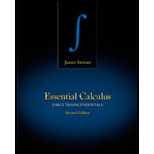# To interpret: About the function f which has critical point at (1, 1) with continuous second derivatives and f x x ( 1 , 1 ) = 4 , f x y ( 1 , 1 ) = 1 and f y y ( 1 , 1 ) = 2 .### Essential Calculus: Early Transcen...

2nd Edition
James Stewart
Publisher: Cengage Learning
ISBN: 9781133112280### Essential Calculus: Early Transcen...

2nd Edition
James Stewart
Publisher: Cengage Learning
ISBN: 9781133112280

#### Solutions

Chapter 11.7, Problem 1E

(a)

To determine

## To interpret: About the function f which has critical point at (1, 1) with continuous second derivatives and fxx(1,1)=4 , fxy(1,1)=1 and fyy(1,1)=2 .

(b)

To determine

### Want to see the full answer?

Check out a sample textbook solution.

### Want to see this answer and more?

Experts are waiting 24/7 to provide step-by-step solutions in as fast as 30 minutes!*

*Response times may vary by subject and question complexity. Median response time is 34 minutes for paid subscribers and may be longer for promotional offers.